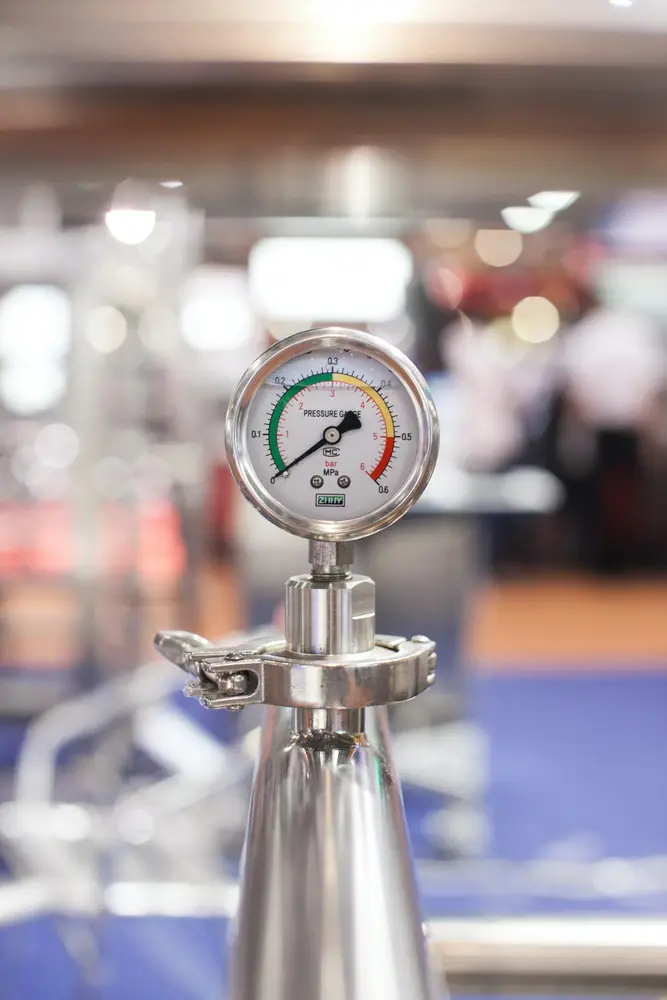# Pressure

Pressure is the amount of force acting upon a particular area of an object.

• The formula for calculating the pressure on an object is:
• Pressure (N/m2) = Force (N) / Area (m2)
• The unit N/m2 is known as Pascals.
• This formula shows that by decreasing the area you will increase the pressure e.g. knives need to exert a big pressure so they have a very narrow area.
• Pressure in solids. The pressure only acts in one direction in solids.
• Pressure in liquids. The pressure in liquid gets higher as you go down. The pressure of liquids acts in all directions not just down.
• Pressure in gases. Gas molecules are free to move anywhere therefore pressure of gases acts in all directions. The pressure gets higher as you go down because of the weight of the gas (air).
Forces and motion

#### Acceleration

##### Acceleration

Forces and motion

#### Forces

##### Forces

Forces and motion

#### Forumulae

##### Forumulae

Forces and motion

#### Hooke's Law

##### Hooke's Law

Forces and motion

#### Momentum

##### Momentum

Forces and motion